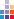# What is an armstrong number?

UnCategorised, Sachin Puri, 2012-05-24 20:26:47

An Armstrong number is a n digit number, which is equal to the sum of the nth powers of its digits.

All the 1 digit numbers (1-9) are Armstrong number because

• 1*1=1 which is equals to number (1) itself,
• 2*1=2 which is equals to number(2) itself so on for all the 1 digit numbers (1-9).

There are no 2 digit Armstrong numbers.

An Armstrong number of three digit is a number such that that sum of the cubes of it's digits is equal to the number itself. so to check if a 3 digit number is an armstrong number or not we have to multiply all the three digits with itself 3 times.

For example 153 is an Armstrong number because cube of 1 is 1(1x1x1=1) + cube of 5 is 125(5*5*5=125) + cube of 3 is 27(3*3*3=27). Now add all the cubes 1+125+27=153 which is equals to number itself

### Example 1: Check if 153 is an armstrong number or not

There are three digits in 153. First Digit is 1, Second digit is 5, and Third digit is 3.

Now,

We will multiply each of these three digits i.e 1,5,3 with itself 3 times because there are 3 digits in 153

So,

```1 * 1 * 1 =   1

5 * 5 * 5 = 125

3 * 3 * 3 =  27
___________________
Sum of=  153
```

Because the sum is equals to the digit itself, so we can say that 153 is an armstrong number.

### Example 2: Check if 154 is an armstrong number or not

```1 * 1 * 1 =   1

5 * 5 * 5 = 125

4 * 4 * 4 =  64
___________________
Sum of=  190

```

So in this example we can see that when we multiply each digit of 154 (i.e. 1 and 5 and 4) with itself 3 times (because 153 has three digits) we get these three numbers (1 and 125 and 64) and then we add these numbers i.e 1+125+64 we get 190

Because the sum 190 is not equals to the digit (154) itself, so we can say that 154 is not an armstrong number.

### Example 3: Check if 370 is an armstrong number or not

```3 * 3 * 3 =  27

7 * 7 * 7 = 343

0 * 0 * 0 =   0
___________________
Sum of=  370

```

So in this example we can see that when we multiply each digit of 370 (i.e 3 and 4 and 0) with itself 3 times (because 370 has three digits) we get these three numbers (27 and 343 and 0) and then we add these numbers i.e 27+343+0 we get 370

Because the sum 370 is equals to the digit 370 itself, so we can say that 370 is an armstrong number.

### Some Exercises for you:

Check if these are armstrong numbers or not,

1. 223
2. 371
3. 402
4. 407by magzine post news on 28-Feb-2019 07:13 pm
Hey There. I found your blog using msn. This is a very well written article. I’ll be sure to bookmark it and come back to read more of your useful info. Thanks for the post. I’ll definitely return.
•  0 Like
•  0 Dislike
• Reportby Vishnu Shukla on 31-Oct-2015 03:44 pm
C program to print Armstrong numbers from 1 to 500 #include int main(){ int num,r,sum,temp; for(num=1;num<=500;num++){ temp=num; sum = 0; while(temp!=0){ r=temp%10; temp=temp/10; sum=sum+(r*r*r); } if(sum==num) printf("%d ",num); } return 0; } Output: 1 153 370 371 407
•  4 Like
•  2 Dislike
• Reportby Vishnu Shukla on 31-Oct-2015 03:42 pm
C program to print Armstrong numbers from 1 to 500 #include int main(){ int num,r,sum,temp; for(num=1;num<=500;num++){ temp=num; sum = 0; while(temp!=0){ r=temp%10; temp=temp/10; sum=sum+(r*r*r); } if(sum==num) printf("%d ",num); } return 0; } Output: 1 153 370 371 407
•  3 Like
•  2 Dislike
• Reportby Manohar kumar bishwas on 27-Sep-2015 02:05 am
Supper explanation
•  2 Like
•  2 Dislike
• Reportby saini g on 12-Jun-2015 07:04 pm
that's a enough info about armstrong numbers (and i m not satisfied with this
•  2 Like
•  2 Dislike
• Reportby Ruchi Udani on 05-Apr-2015 06:20 pm
what is the common equation of armstrong number?
•  2 Like
•  2 Dislike
• Reportby ohk on 06-Jan-2015 01:12 pm
nyc
•  2 Like
•  2 Dislike
• Reportby pandiyarajan on 04-Nov-2014 12:36 pm
•  2 Like
•  2 Dislike
• Reportby Rajeev on 11-Sep-2013 10:14 pm
Best explanation
•  3 Like
•  3 Dislike
• Reportby shashidhar on 03-Apr-2013 08:58 pm
why we consider 1 as armstrong no in 3 digit
•  5 Like
•  3 Dislike
• Reportby Manish Kumar on 22-Apr-2013 12:02 am
We don't consider 1 as armstrong no is 3 digit. If we are calculating armstrong number of three digits we will consider only those numbers which have 3 digits in it.
•  3 Like
•  3 Dislike
• Reportby pooja on 30-Sep-2012 11:30 pm
thank you vvery much
•  3 Like
•  3 Dislike
• Reportby azam on 07-Sep-2012 09:35 am
thnks sir i want to recieve some moresolutionsHi, I am Sachin Puri, I am a Programmer. Programming is my work and it is the thing i like to do most in my free time.